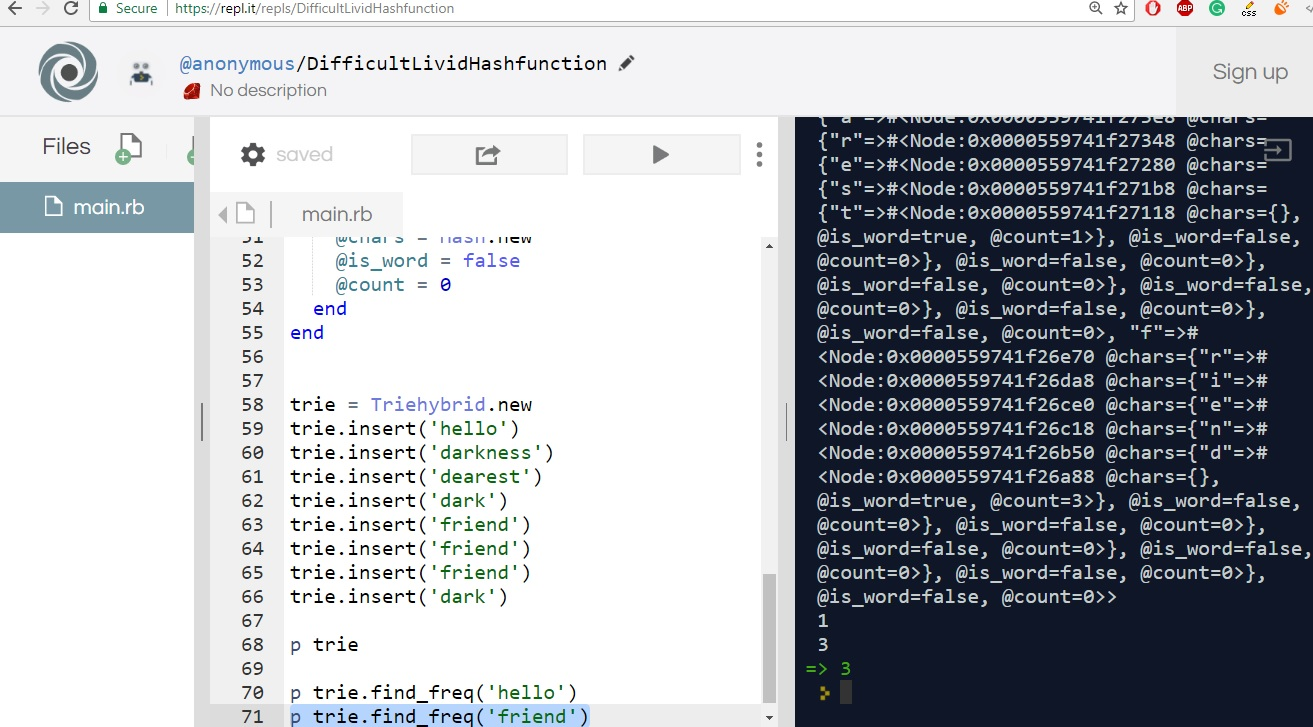# DESIGN A METHOD TO FIND THE FREQUENCY OF OCCURRENCES OF ANY GIVEN WORD IN A BOOK. WHAT IS WE WERE RUNNING THE ALGO MULTIPLE TIMES | TRIE | CRACKING THE CODING INTERVIEW | CTCI | PROGRAMMING | INTERVIEW

By: Saurav

2018-04-06 03:51:00 UTC

Cracking the coding interview recommends the use of a hash here.Performance wise they are both similar. The only thing Trie excels at is the space complexity.

Here, let's see the use of a hybrid trie to handle this problem

```class Triehybrid
def initialize
@root = Node.new
end

def insert(word)
node = @root
i = 0
while i < word.length
if node.chars.key?(word[i])
node = node.chars[word[i]]
i += 1
else
break
end
end

while i < word.length
node.chars[word[i]] = Node.new
node = node.chars[word[i]]
i += 1
end

node.is_word = true
node.count += 1

return @root
end

def find_freq(word)
node = @root
(0..word.length-1).each do |i|
if node.chars.key?(word[i])
node = node.chars[word[i]]
else
return -1
end
end

node.is_word ? node.count : -1
end

end

class Node
attr_accessor :chars, :is_word, :count

def initialize
@chars = Hash.new
@is_word = false
@count = 0
end
end ```

```trie = Triehybrid.new
trie.insert('hello')
trie.insert('darkness')
trie.insert('dearest')
trie.insert('dark')
trie.insert('friend')
trie.insert('friend')
trie.insert('friend')
trie.insert('dark')

p trie

p trie.find_freq('hello')
p trie.find_freq('friend')
```

As we see below, our code works fine and it's easy to search the frequency of the word through the API.### Let me know what you think! twitter: sprakash24octlinkedin

Owned & Maintained by Saurav Prakash

If you like what you see, you can help me cover server costs or buy me a cup of coffee though donation :)